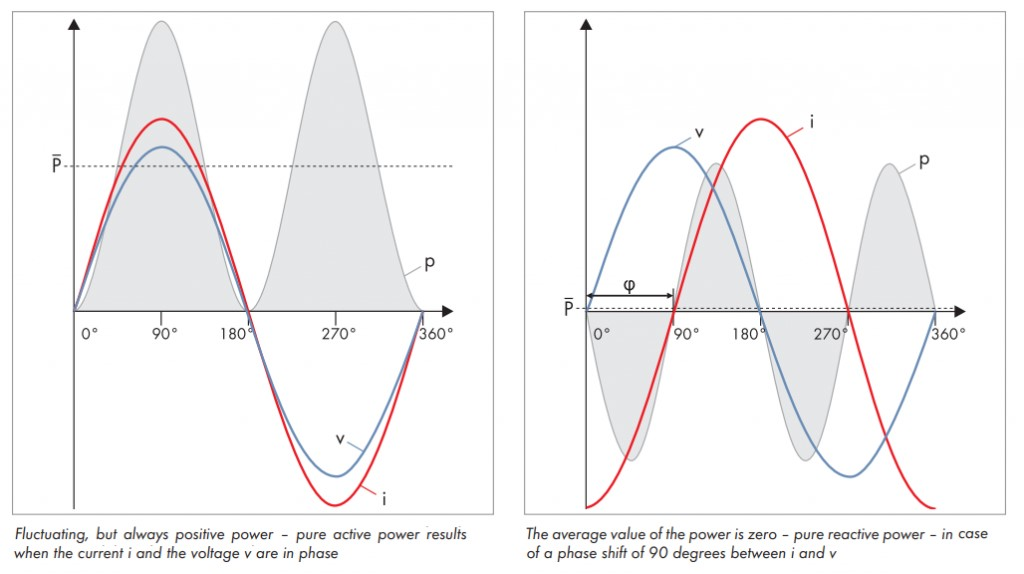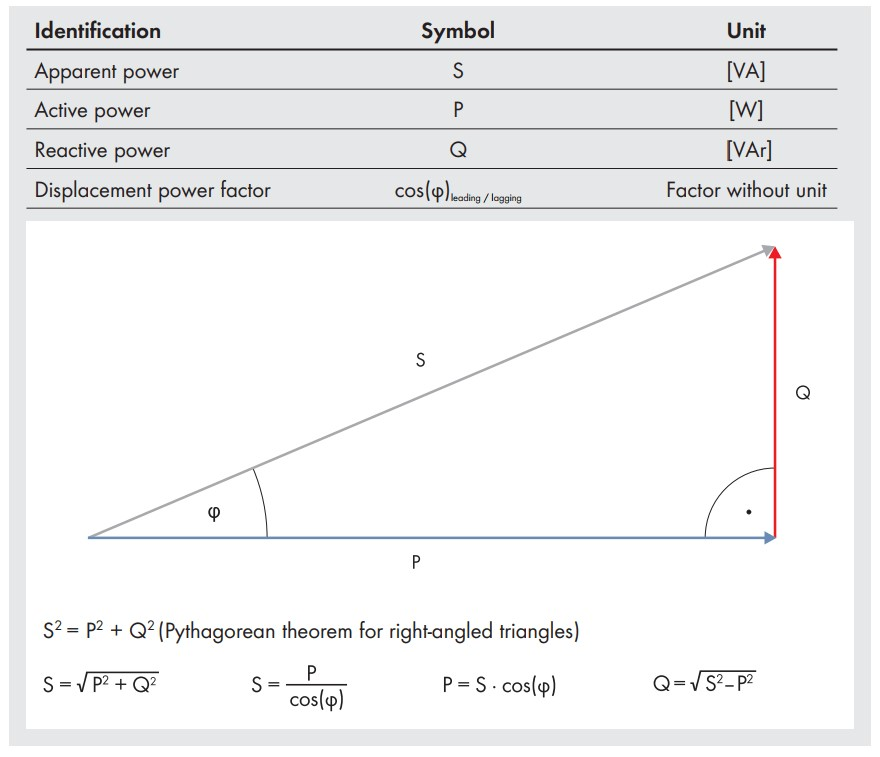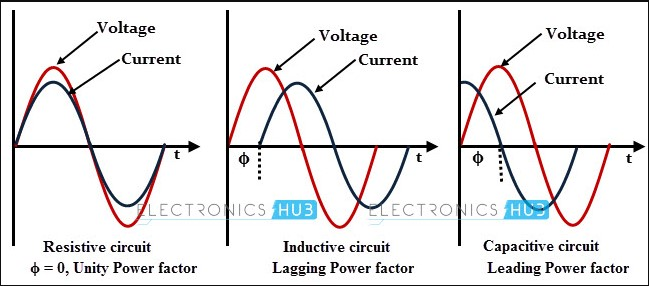# Understand the Power Factor

## Understand the Power Factor

### What is power factor?

Power factor is defined as the cosine angle between voltage and current in an alternating current (AC) circuit.

A phase angle difference, ϕ exists between voltage and currents in an AC circuit. The cosine of ϕ (or Cos ϕ) is termed as the power factor.

Alternating current, put simply, voltage and current that are transmitted throughout the electric power grid in a sinusoidal waveform averaging 0. When these current and voltage waveforms are perfectly synchronized in time, they have a power factor of 1 or pure active power.Example of pure active power (left) with current and voltage perfectly in-phase, and of pure reactive power (right) with current and voltage perfectly out-of-phase.

When we consume electricity (in pumps, fridges, lights, etc) current and voltage waveforms can go out of alignment. This will lead to a power factor ≠ 1. As a site’s power factor moves further away from 1, they will typically incur increased grid quality supply charges from their electricity provider.

### What is ACTIVE, REACTIVE and APPARENT power?

We also need to understand the relationship between ACTIVE, REACTIVE and APPARENT power. Apparent power consists of active and reactive power, two different types of power existing only in its pure nature. Because active and reactive power don’t have a relationship, it is impossible to convert one power type to the other. A graphical model of such relationship is a cartesian coordinate system with active power for the x-axis and reactive power in the y-axis. These 3 power types are related together according to Pythagoras’ theorem as shown in the following diagram.Relationship between Apparent, Active and Reactive power.

### What is Resistive, Inductive and Capacitive circuit?

If the circuit consists of inductive elements or it behaves like an inductive circuit (where current lag behind the voltage), then the power factor in that circuit is referred as a lagging power factor.

On the other hand, if the circuit is capacitive in nature where current leads the voltage, then the power factor is referred as the leading power factor. If there is no phase angle difference between the voltage and current then it is unity power factor.